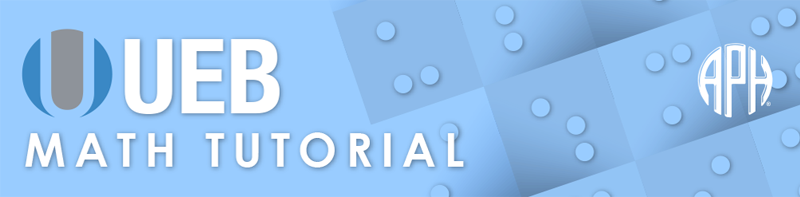Practice Problems -   -  Use 6 Dot Entry   Switch to Nemeth Tutorial

# Lesson 7.0: Set Notation: Complements and Subsets

## Symbols

$\in \phantom{\rule{.3em}{0ex}}\text{is an element of}$
⠘⠑

$\ni \phantom{\rule{.3em}{0ex}}\text{contains an element of}$
⠈⠘⠑

$\subset \phantom{\rule{.3em}{0ex}}\text{contained in}$
⠘⠣

$\subseteq \phantom{\rule{.3em}{0ex}}\text{contained in or equal to}$
⠸⠘⠣

$\supset \phantom{\rule{.3em}{0ex}}\text{contains}$
⠘⠜

$\supseteq \phantom{\rule{.3em}{0ex}}\text{contains or equal to}$
⠸⠘⠜

$\varnothing \phantom{\rule{.3em}{0ex}}\text{null set}$
⠈⠚

## Review

In previous lessons, it was noted that the empty set may be represented by an empty set of braces facing each other, {}. It is also known as the null set or ∅.

A letter or unbroken sequence of letters is standing alone if the symbols before and after the letter or sequence are spaces, hyphens, dashes or any combination, or if on both sides the only intervening symbols between the letter or sequence and space, hyphen, or dash are common literary punctuation or indicator symbols. [Rules of Unified English Braille §2.6] A braille symbol that is standing alone can have a contraction (grade 2) meaning. When the grade 1 meaning is desired, grade 1 mode must be turned on.

Follow print for spacing and punctuation of brackets and braces. The numeric indicator must be used with a digit that immediately follows a bracket or brace since both of these symbols terminate numeric mode.

## Explanation

A set is a collection of objects that is defined as having a certain property in common. An object that is part of a set is referred to as an element or member of the set. In set notation, each element of the set is listed separated by a comma, with all of the elements between curly brackets or braces. Curly brackets were introduced in an earlier lesson. Follow print for spacing of symbols.

### Example 1

$\left\{2,4,6\right\}$
⠸⠣⠼⠃⠂⠀⠼⠙⠂⠀⠼⠋⠸⠜

An element can be identified as belonging to a set by using the "is an element of" symbol ∈, (variant epsilon). The variant epsilon is a two cell symbol in braille, dots four five in the first cell and dots one five in the second cell.

### Example 2

$4\in \left\{2,4,6\right\}$
⠼⠙⠀⠘⠑⠀⠸⠣⠼⠃⠂⠀⠼⠙⠂⠀⠼⠋⠸⠜

Sets are typically named using capital letters. The complement, or objects that are not in a specified set, are often written in print with the same upper-case letter followed by the prime symbol. For example, the complement of set A would be written as A′. The symbol for prime in braille, dots two three five six, was introduced in an earlier lesson.

### Example 3

$\text{The complement to}\phantom{\rule{.3em}{0ex}}B\phantom{\rule{.3em}{0ex}}\text{is}\phantom{\rule{.3em}{0ex}}{B}^{\prime }\text{.}$
⠠⠮⠀⠉⠕⠍⠏⠇⠑⠰⠞⠀⠞⠕⠀⠰⠠⠃⠀⠊⠎⠀⠠⠃⠰⠶⠲

### Example 4

$\text{If}\phantom{\rule{.3em}{0ex}}A=\left\{2,4,6\right\}\text{, what is}\phantom{\rule{.3em}{0ex}}{A}^{\prime }\text{?}$
⠠⠊⠋⠀⠠⠁⠀⠐⠶⠀⠸⠣⠼⠃⠂⠀⠼⠙⠂⠀⠼⠋⠸⠜⠂⠀⠱⠁⠞⠀⠊⠎⠀⠠⠁⠰⠶⠦

### Example 5

$4\in A$
⠼⠙⠀⠘⠑⠀⠠⠁

In Example 6, the expression indicates that 4 is not an element of set A by placing a slash through the variant epsilon symbol. The line through previous item indicator is placed immediately after the variant epsilon in braille.

### Example 6

$4\notin A$
⠼⠙⠀⠘⠑⠈⠱⠀⠠⠁

Symbols are commonly used to describe the relationship of one set to another. Pieces of a set that are included within another set are known as a subset. A subset has some or all elements of the other set. A proper subset contains some elements of the set but not all. The symbol for a proper subset, ⊂ (contained in), is two cells in braille: dots four five in the first cell and dots one two six in the second cell. The symbol for a subset (contained in or equal to) ⊆, is the subset symbol with a horizontal line displayed beneath it. The symbol for contained in or equal to is three cells; dots four five six in the first cell, dots four five in the second cell, and dots one two six in the third cell.

### Example 7

$A\subseteq B$
⠠⠁⠀⠸⠘⠣⠀⠰⠠⠃

The empty or null set denoted by ∅, is also a subset of any given set. It is always a proper subset of any set except itself. The symbol is made with a dot four prefix and dots two four five in the root cell.

### Example 8

$\varnothing \subseteq A$
⠈⠚⠀⠸⠘⠣⠀⠠⠁

### Example 9

$A\subset B$
⠠⠁⠀⠘⠣⠀⠰⠠⠃

A superset has the same elements as the other set, or more. The symbol for inclusion, superset, is ⊃. The inclusion superset symbol is two cells: dots four five, three four five. A proper superset has all of the elements of the other set and more. In print, the symbol for a proper superset is the superset symbol with a horizontal line displayed beneath it, ⊇ (contains or equal to). The proper superset symbol is three cells: dots four five six in the first cell, dots four five in the second cell, and dots three four five in the third cell.

### Example 10

$A\supset C$
⠠⠁⠀⠘⠜⠀⠰⠠⠉

### Example 11

$A\supseteq C$
⠠⠁⠀⠸⠘⠜⠀⠰⠠⠉

In Example 12, the symbol for "contained in" is shown above the equals sign in print. The vertical juxtaposition indicator (introduced in a previous lesson) is required in braille. Note that this symbol also has a grade 2 meaning, therefore the grade 1 indicator is required.

### Example 12

$A⫅B$
⠠⠁⠀⠘⠣⠰⠻⠐⠶⠀⠰⠠⠃